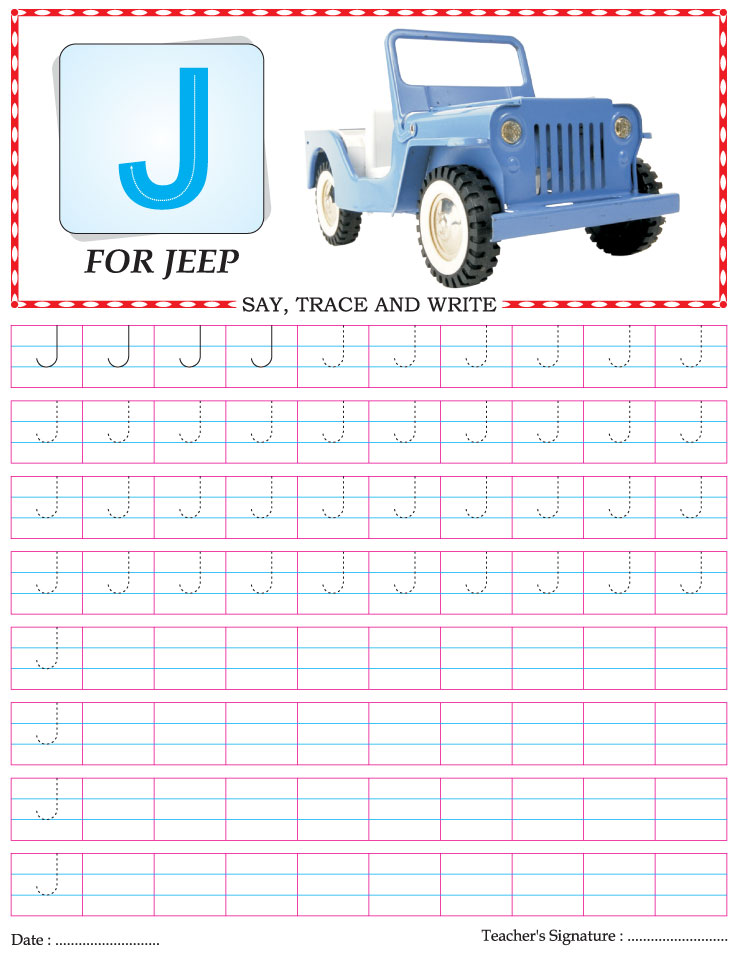# Home

## Worksheet Category

### Capital letter writing practice worksheet alphabet J

Page of 26Page of 26Capital letter writing practice worksheet alphabet ZCapital letter writing practice worksheet alphabet YCapital letter writing practice worksheet alphabet XCapital letter writing practice worksheet alphabet WCapital letter writing practice worksheet alphabet VCapital letter writing practice worksheet alphabet UCapital letter writing practice worksheet alphabet TCapital letter writing practice worksheet alphabet SCapital letter writing practice worksheet alphabet RCapital letter writing practice worksheet alphabet QCapital letter writing practice worksheet alphabet PCapital letter writing practice worksheet alphabet OCapital letter writing practice worksheet alphabet NCapital letter writing practice worksheet alphabet MCapital letter writing practice worksheet alphabet LCapital letter writing practice worksheet alphabet KCapital letter writing practice worksheet alphabet ICapital letter writing practice worksheet alphabet HCapital letter writing practice worksheet alphabet GCapital letter writing practice worksheet alphabet FCapital letter writing practice worksheet alphabet ECapital letter writing practice worksheet alphabet DCapital letter writing practice worksheet alphabet CCapital letter writing practice worksheet alphabet BCapital letter writing practice worksheet alphabet A